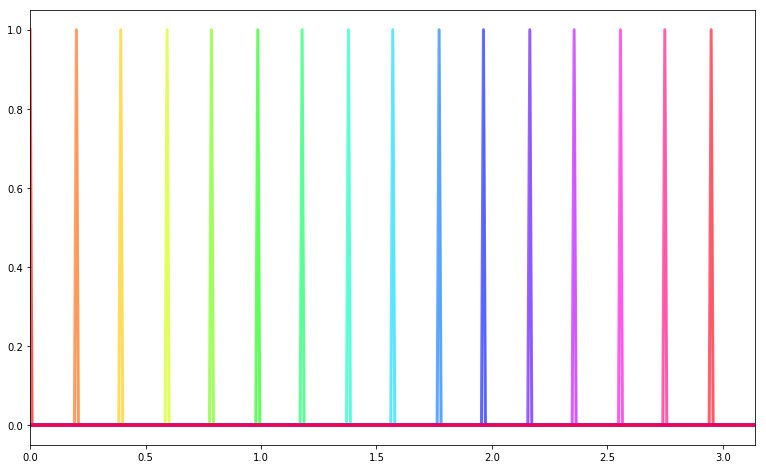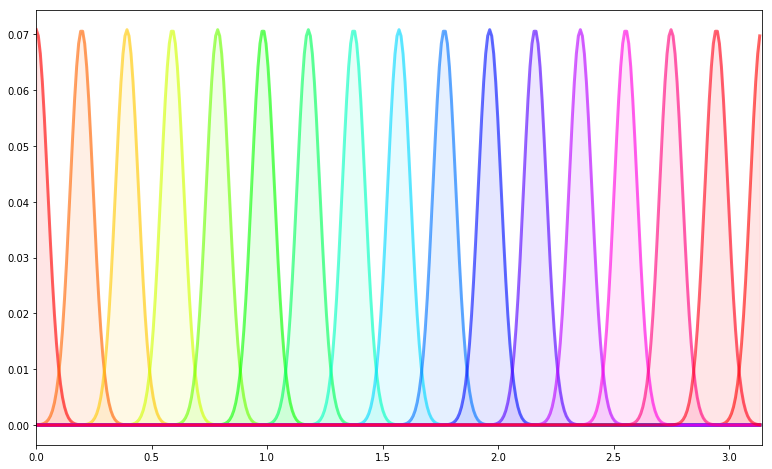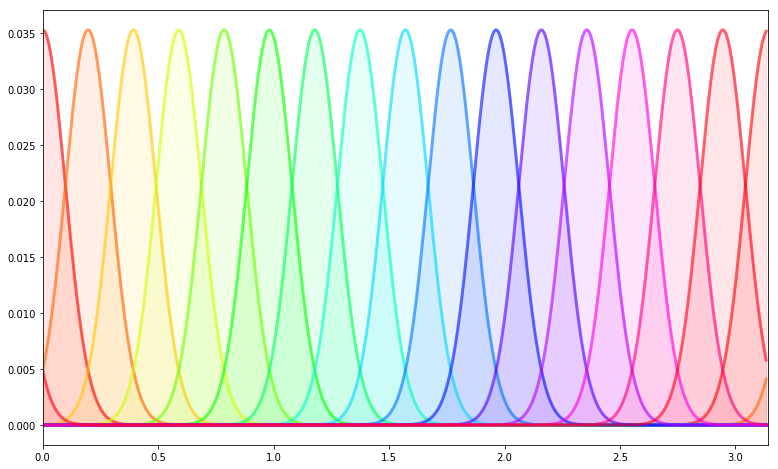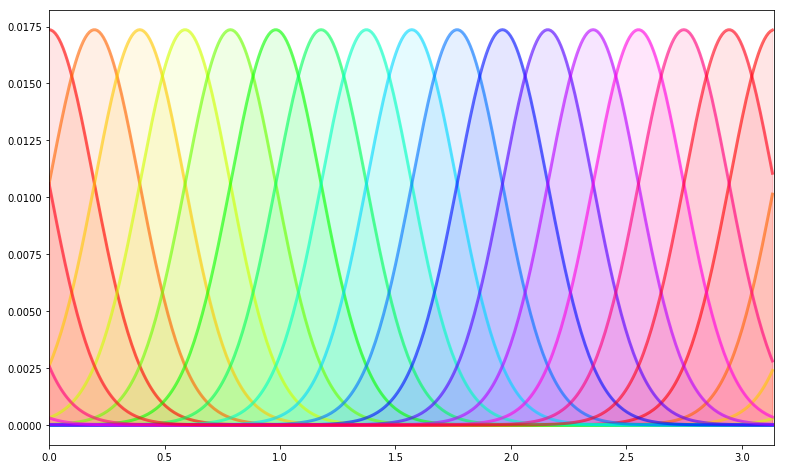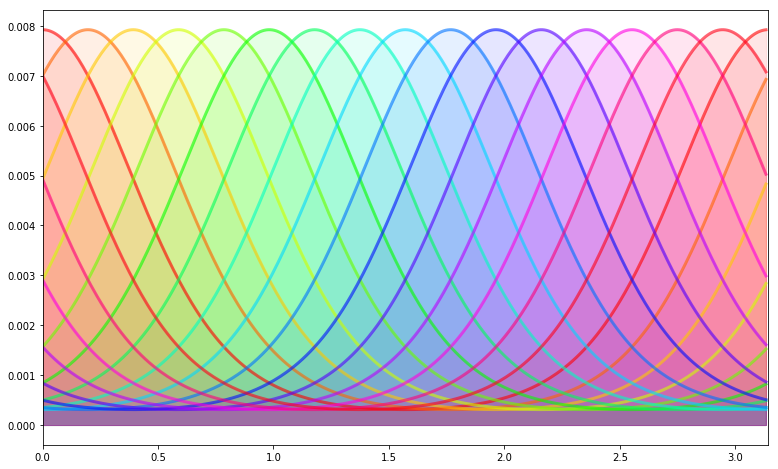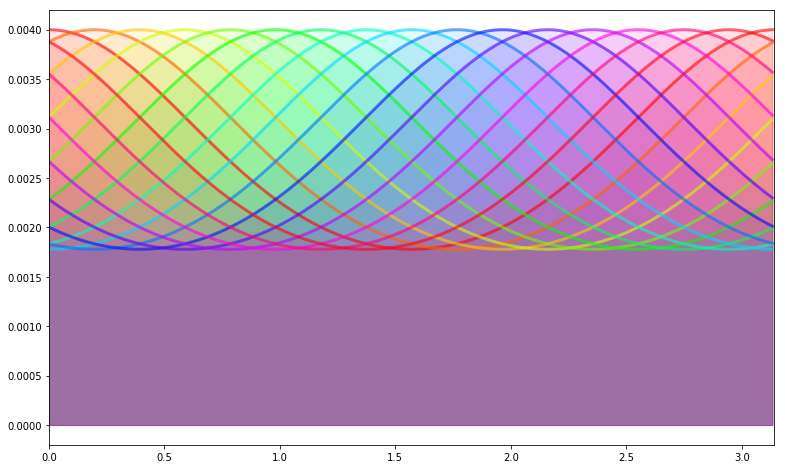# Recruiting different population ratios in V1 using orientation components: defining a protocol

A feature of MotionClouds is the ability to precisely tune the precision of information following the principal axes. One which is particularly relevant for the primary visual cortical area of primates (area V1) is to tune the otirentation mean and bandwidth.

This is part of a larger study to tune orientation bandwidth.

### summary of the electro-physiology protocol¶

In :
import os
import numpy as np
import MotionClouds as mc
downscale = 1
fx, fy, ft = mc.get_grids(mc.N_X/downscale, mc.N_Y/downscale, mc.N_frame/downscale)

name = 'balaV1'
mc.figpath = os.path.join('../files', '2014-11-10_balaV1-protocol')
if not(os.path.isdir(mc.figpath)): os.mkdir(mc.figpath)

In :
N_X = fx.shape
width = 29.7*256/1050
sf_0 = 4.*width/N_X
B_V = 2.5     # BW temporal frequency (speed plane thickness)
B_sf = sf_0   # BW spatial frequency
theta = 0.0   # Central orientation
B_theta_low, B_theta_high = np.pi/32, 2*np.pi
B_V = 0.5
seed=12234565

mc1 = mc.envelope_gabor(fx, fy, ft, V_X=0., V_Y=0., B_V=B_V, sf_0=sf_0, B_sf=B_sf, theta=theta, B_theta=B_theta_low)
mc2 = mc.envelope_gabor(fx, fy, ft, V_X=0., V_Y=0., B_V=B_V, sf_0=sf_0, B_sf=B_sf, theta=theta, B_theta=B_theta_high)
name_ = name + '_1'
mc.figures(mc1, name_, seed=seed, figpath=mc.figpath)
mc.in_show_video(name_, figpath=mc.figpath)
name_ = name + '_2'
mc.figures(mc2, name_, seed=seed, figpath=mc.figpath)
mc.in_show_video(name_, figpath=mc.figpath)

/usr/local/lib/python3.7/site-packages/vispy/visuals/isocurve.py:22: UserWarning: VisPy is not yet compatible with matplotlib 2.2+
warnings.warn("VisPy is not yet compatible with matplotlib 2.2+")

Failed to generate the cube: list index out of range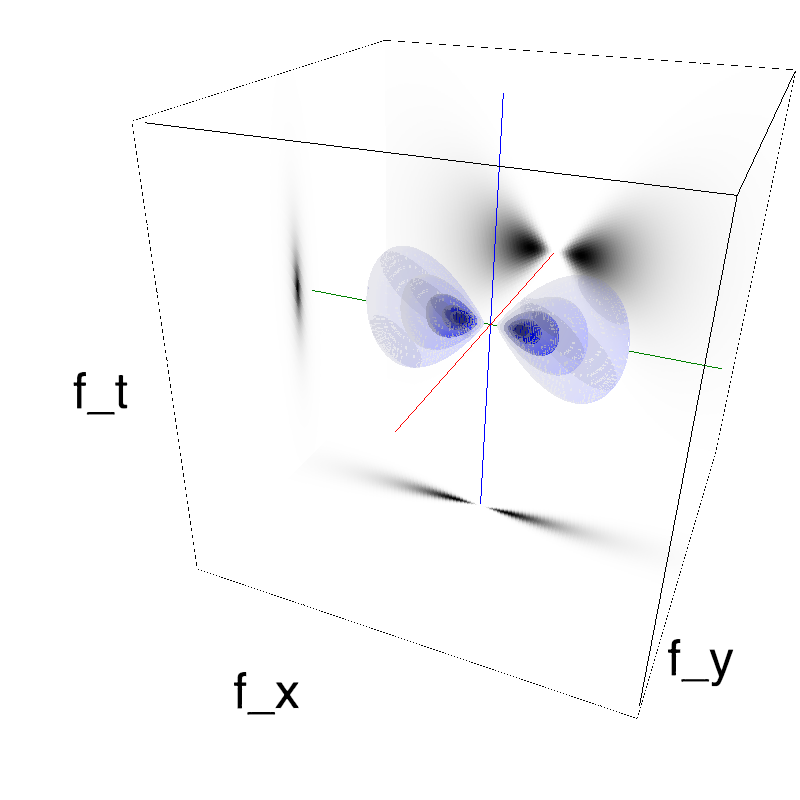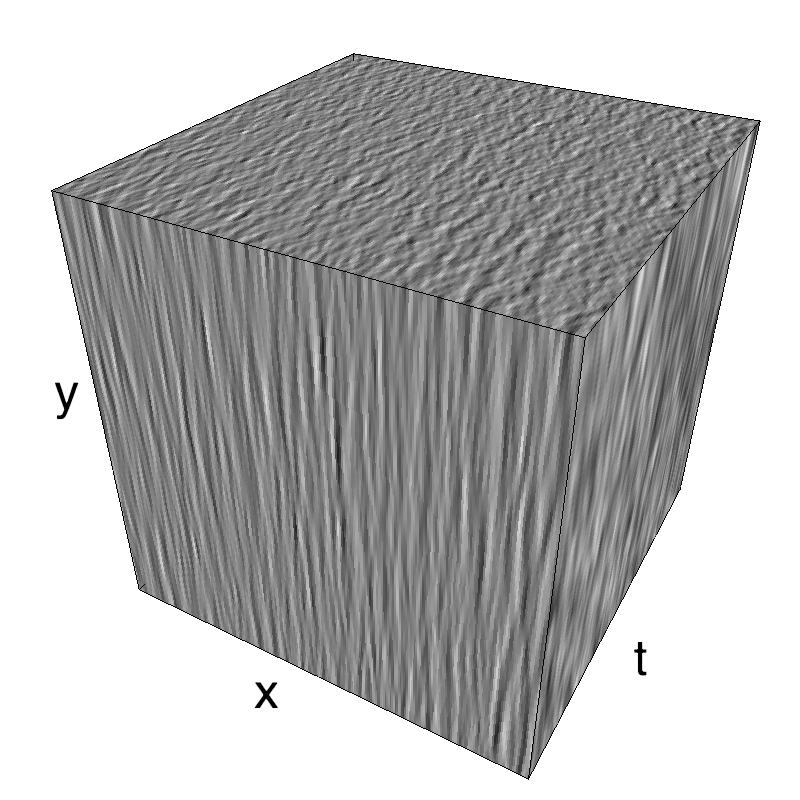Failed to generate the visualisation: list index out of range
Failed to generate the cube: list index out of range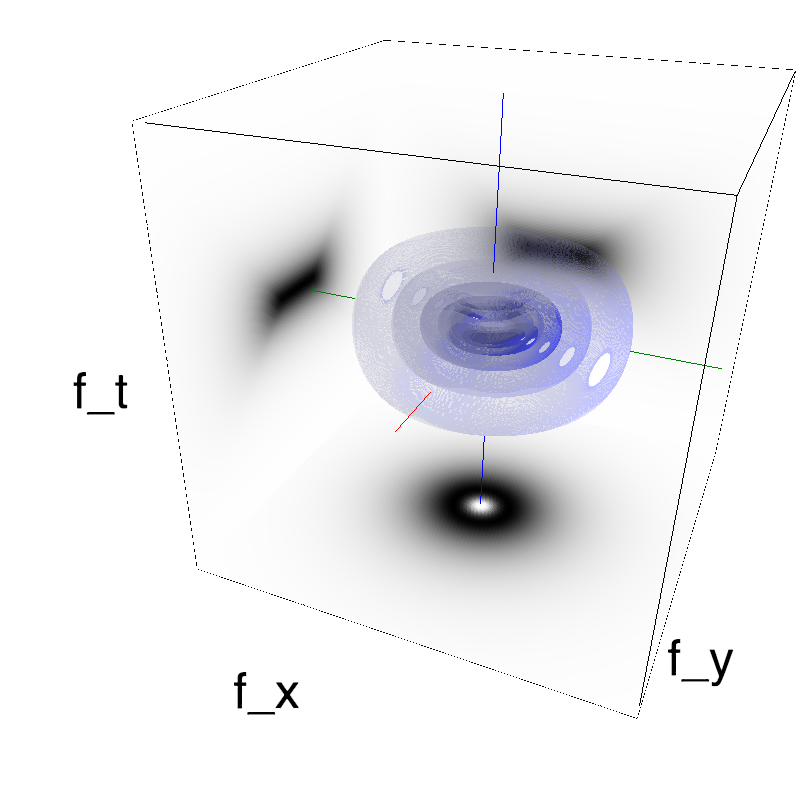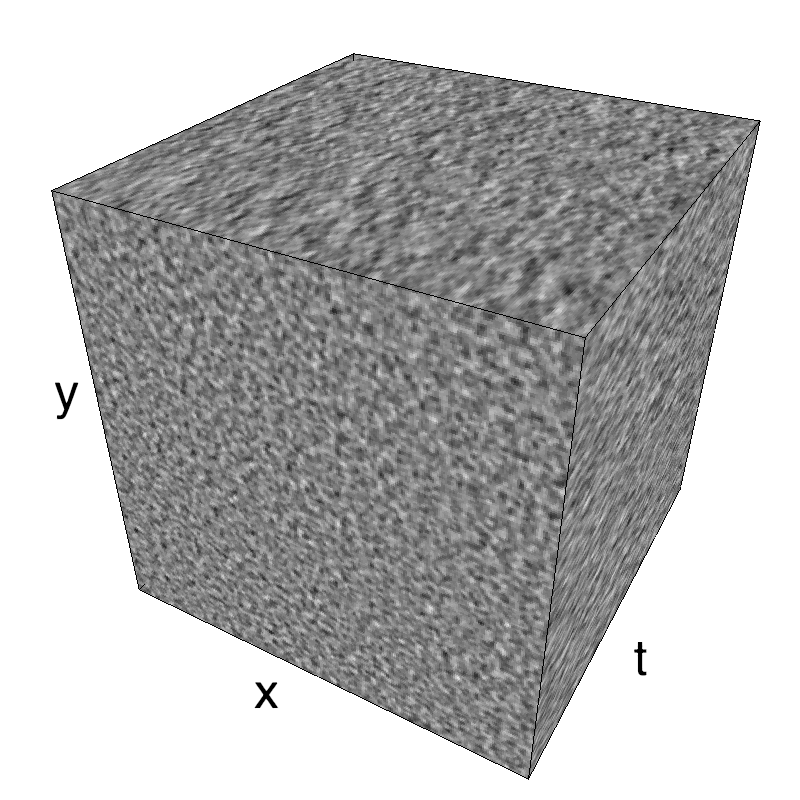This figure shows how one can create different MotionCloud stimuli that specifically target different population in V1. We show in the two lines of this table motion cloud component with a (Top) narrow orientation bandwith (Bottom) a wide bandwitdh: perceptually, there is no predominant position or speed, just different orientation contents.
Columns represent isometric projections of a cube. The left column displays iso-surfaces of the spectral envelope by displaying enclosing volumes at 5 different energy values with respect to the peak amplitude of the Fourier spectrum.
The middle column shows an isometric view of the faces of the movie cube. The first frame of the movie lies on the x-y plane, the x-t plane lies on the top face and motion direction is seen as diagonal lines on this face (vertical motion is similarly see in the y-t face). The third column displays the actual movie as an animation.

Note: an analysis of the response of a neural network to such stimulations as been explored by Chloé Pasturel.

In :
from __future__ import division, print_function
%matplotlib inline
import matplotlib.pyplot as plt
import numpy as np
np.set_printoptions(precision=2, suppress=True)


### designing a protocol¶

In light with the classical result that OS in V1 should be invariant to contrast (of course in a limited range) and that contrast is a variable manipulating the relative amount of extrinsic noise (that is, originating from the source, external to the system), it is is crucial to titrate the effect of the level of intrinsic noise by changing the precision of the variable that is coded in V1.

• is precision (here, of orientation) coded in the neural activity?
• does a balanced network account for that effect?

Designing an experiment is mainly constrained by the time available for recording and we will estimate it here (optimistically) to 3 hours. The protocal consists in showing a set of motion clouds with diferent parameters. Spatial frequency will be tuned from the litterature for the given eccentricity in V1, while average speed (as in the above example) is always nil. The remaining parameters are the precision in spatial frequency ($B_f$), the precision in time ($B_V$) which is inversely proportional to the average life-time of the textons, orientation $\theta$ and precision for orientation ($B_\theta$). To allow a comparison with the classical grating, we will start from infinitely fine envelopes (Dirac) with $B_\theta=0$, $B_V=0$ and $B_f=0$ and then grow from this reference point.

The main goal is to study OS as a function of orientation bandwidth, so we will test 16 orientations (including the cardinals). Then, we choose 5 levels of precision for orientation ($B_\theta$). The following plot shows how we cover the orientation space with 16 orientations and an increasing bandwidth:

In :
def envelope(th, theta, B_theta):
if B_theta==np.inf:
env = np.ones_like(th)
elif B_theta==0:
env = np.zeros_like(th)
env[np.argmin(th < theta)] = 1.
else:
env = np.exp((np.cos(2*(th-theta))-1)/4/B_theta**2)
return env/env.sum()

In :
N_theta = 16
B_theta = np.pi/16
bins = 360
th = np.linspace(0, np.pi, bins, endpoint=False)
for B_theta_ in [0, np.pi/64, np.pi/32, np.pi/16, np.pi/8, np.pi/4, np.pi/2, np.inf]:
fig, ax = plt.subplots(1, 1, figsize=(13, 8))
for theta, color in zip(np.linspace(0, np.pi, N_theta, endpoint=False),
[plt.cm.hsv(h) for h in np.linspace(0, 1, N_theta)]):
ax.plot(th, envelope(th, theta, B_theta_), alpha=.6, color=color, lw=3)
ax.fill_between(th, 0, envelope(th, theta, B_theta_), alpha=.1, color=color)
ax.set_xlim([0, np.pi])